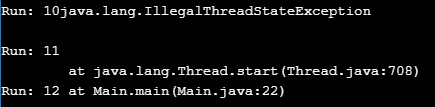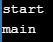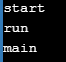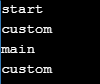##### Question: Can we call the run method directly from the Main method?

Yes, we can call the run method directly. If we call it directly then a user-defined thread in the java stack area is not created. It is executed in the main thread.

```public class MyThread extends Thread
{
public void run ()
{
for (int i = 0; i <= 50; i++)
{
System.out.println ("Run: " + i);
}
}
public static void main (String[]args)
{
mt.run ();
for (int i = 0; i <= 50; i++)
{
System.out.println ("Main: " + i);
}
}
}```

Output: main method and run method executed in the same main thread sequentially. Since the run method is called before the main method for loop, the first-run method for loop output is printed then later the main method for loop output is printed.

##### Question: What will be the output in the below program?

Here, we are calling both the run and start methods. Run method logic is executed two times. It is executed in the main thread since it is directly called from the main method; also it is executed in the customs thread due to the start method call.

```public class Main extends Thread
{
public void run ()
{
for (int i = 0; i <= 50; i++)
{
System.out.println ("Run: " + i);
}
}
public static void main (String[]args)
{
Main mt = new Main();
mt.run();
mt.start();
for (int i = 0; i <= 50; i++)
{
System.out.println ("Main: " + i);
}
}
}```

Output: first run method in the main thread completes its execution, then due to the start method call user-defined thread is created and the run method is also again. Now run method in the user-defined thread and the main method for loop in the main thread are executed concurrently.

##### Question: What will be the output in the below program?

Here, we call the start() before the run() method. Run method logic is executed concurrently, one is from thread-0 and another is from the main thread. After completion of the run method execution in the main thread main method for loop is started.

```public class Main extends Thread
{
public void run ()
{
for (int i = 0; i <= 5; i++)
{
System.out.println ("Run: " + i);
}
}
public static void main (String[]args)
{
Main mt = new Main();
mt.start ();
mt.run ();
for (int i = 0; i <= 5; i++)
{
System.out.println ("Main: " + i);
}
}
}```
##### Question: If the start method is called on the thread class object directly, is the subclass run method executed?

No, the run method is executed from the thread class. The basic point to be remembered is the run method is executed from the current thread object of the start method. So in this case thread object is the current object, so the run method is executed from the thread class.

##### Question: Can we call the start method more than once on the same thread object?

No, it leads to exception java.lang.illegalThreadStateException. In the current thread-the thread that calls the start method- the exception is terminated abnormally. See the following example.

```public class Main extends Thread
{
public void run ()
{
for (int i = 0; i <= 50; i++)
{
System.out.println ("Run: " + i);
}
}
public static void main (String[]args)
{
Main mt = new Main();
mt.start ();
mt.start ();
for (int i = 0; i <= 50; i++)
{
System.out.println ("Main: " + i);
}
}
}```

Output: main method execution is terminated means for loop will not be executed. But run method execution in Thread-0 will be continuing. This means that even though main thread execution is terminated custom thread execution is continued.##### Question: How can we create Multiple user-defined Threads in Java Stack Area?

There are two ways to create more than one user-defined thread in the java stacks area

1. Create multiple thread subclass objects and call the start method on each thread object
2. Create multiple subclasses from a thread class, create its object, and call the start method.

In the first approach, all threads execute the same run method logic, because all its thread objects are created from the same class. This approach is recommended only to execute the same logic concurrently with different object states.

```public class MultiThreading extends Thread
{
public void run ()
{
for (int i = 0; i < 50; i++)
{
System.out.println (getName () + "RUN:" + i);
}
}
public static void main (String[]args)
{
mt1.start ();
mt2.start ();
mt3.start ();
}
}```

Note: getName() method returns currently executing thread name.

In the second approach, all threads execute the run method with different logic because thread objects are created from different classes. This is the actual approach used in projects to develop multithreading.

```class AddThread extends Thread
{
int sum = 0;
public void run ()
{
for (int i = 0; i <= 50; i++)
{
sum += i;
System.out.println ("the summation :" + sum);
}
}
}
{
int diff = 0;
public void run ()
{
for (int i = 50; i >= 50; i--)
{
diff -= i;
System.out.println ("the substraction is :" + diff);
}
}
}
public class Main
{
public static void main (String args[])
{
sub.start ();
System.out.println ("main exited");
}
}```
##### Question: Can we override the Start Method?

Yes, we can override it as it is a non-final method. See the following code

```Class MyThread extends Thread
{
Public void run ()
{
System.out.println ("run");
}
Public void start ()
{
System.out.println ("start");
}
}```

When we override the start method custom thread is not created.

##### Question: Then why the Thread class Developer did not declare the start method as Final?

It is a project requirement. Before starting this thread execution if we want to do some validations and calculations to update the current custom thread object state, we should override the start method in subclasses with this validation logic, and then we should start a custom thread. This is the reason thread class developer leaving starts as a non-final method.

##### Question: How can we start Custom Thread From the Overriding method?

We must place super.start() at the end of the overriding method.

```public class MyThread extends Thread
{
public void run ()
{
System.out.println ("run");
}
public void start ()
{
System.out.println ("start");
}
public static void main (String args[])
{
mt.start ();
System.out.println ("main");
}
}```

Output: The custom thread is not created. So run() method is not executed.##### Question: If we call the Run method from the Overriding start method then in which thread the run method is going to be executed?
```public class MyThread extends Thread
{
public void run ()
{
System.out.println ("run");
}
public void start ()
{
System.out.println ("start");
run();
}
public static void main (String args[])
{
mt.start ();
System.out.println ("main");
}
}```

Output: A custom thread is not created, so the run() method is executed in the main thread as the start method is executed in the main thread. So the output will be##### Question: If we call Super.Start in the above program, is custom thread is created, where the run method is executed, and how many times?

Yes, the custom thread is created with the name Thread-0. the run() method is executed two times.

1. In the main thread, because we call it explicitly from the overriding start() method
2. In custom thread because it is implicitly called by JVM because of super.start() call.
```public class MyThread extends Thread
{
public void run ()
{
System.out.println ("custom");
}
public void start ()
{
System.out.println ("start");
run();
super.start ();
}
public static void main (String args[])
{